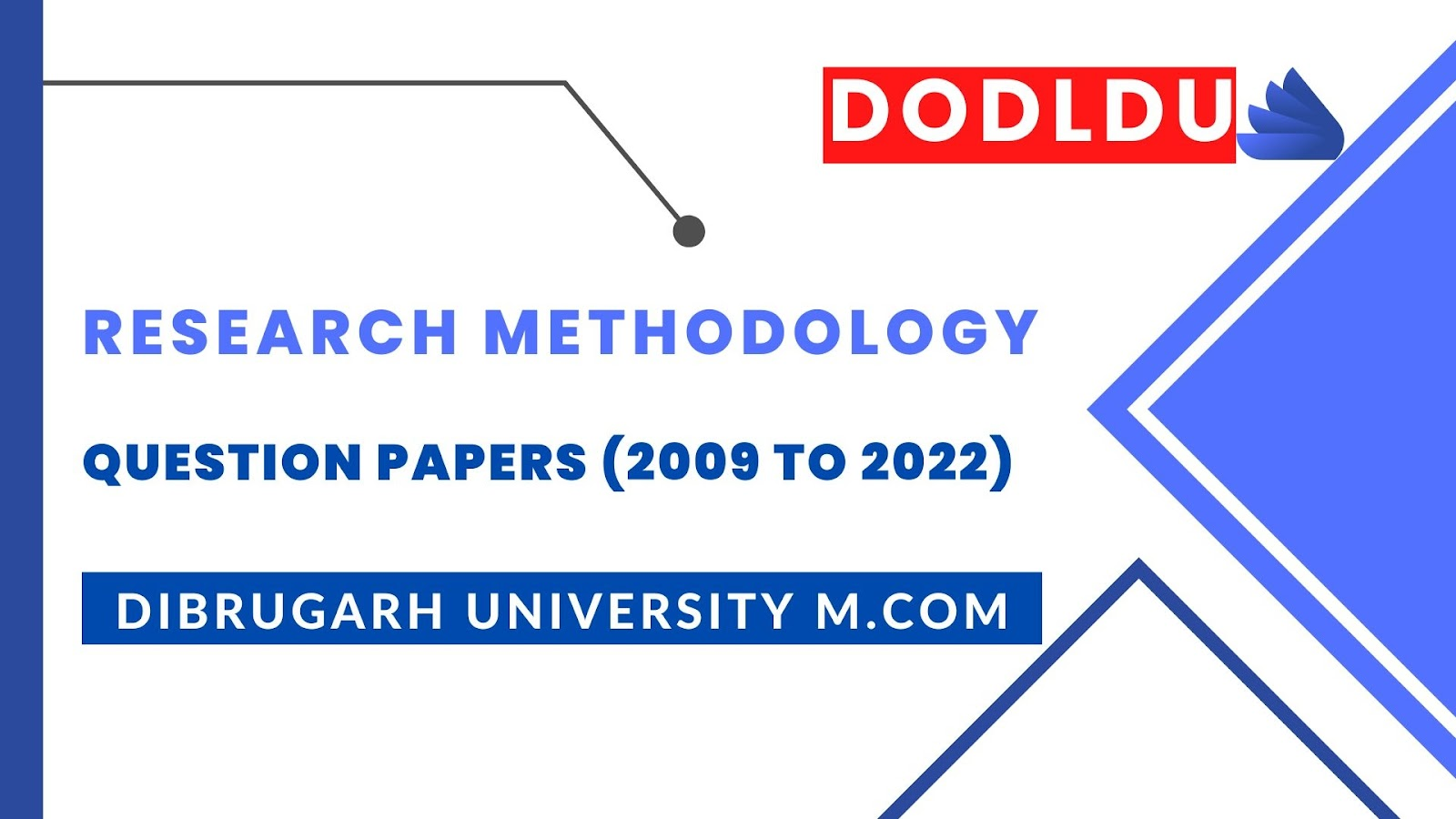# Research Methodology Question Paper 2013 [Dibrugarh University DODLDU M.Com Final Year]## Research Methodology Question Paper 2013 (Nov-Dec)[Dibrugarh University DODLDU M.Com Final Year]

COMMERCE
Paper: 203
(Research Methodology and Statistical Analysis)
Full Marks: 80
Time: 3 hours
The figures in the margin indicate full marks for the questions
1. (a) What is Research Design? Discuss the utility of Research Design.   4+12=16
Or
(b) Write notes on:              4x4=16
1)            Nominal Data.
2)            Ordinal Data.
3)            Interval Data.
4)            Ratio Data.

2. (a) Compare and contrast between:            8x2=16
1)            Measure of Central Tendency Vs. Measure of Variation.
2)            Correlation Vs. Regression Analysis.
Or
(b) What is Time Series Analysis? Find the Trend from the following data:        6+10=16
 Year: Y1 Y2 Y3 Y4 Y5 Y6 Y7 Sales (units): 848 675 513 479 500 425 319

3. (a) Write a note on the characteristics and applications of:   2(4+4)=16
1)            Binomial Distribution.
2)            Poison Distribution.
Or
(b) What is Statistical Decision Theory? Discuss the scope of Statistical Decision Theory in Business.      4+12=16

4. (a) (1) Distinguish between Point and Interval Estimation of Population Mean.    4
(2) Give that size of sample is 100, average height is 64 inches and sample standard deviation is 3 inches. Estimate: Population Limits of (A) Arithmetic Mean, (B) Standard Deviation (C) Variance.            4+4+4=12
Or
 Student Marks Secured In 1st Test Marks Secured In 2nd Test 1 2 3 4 5 6 7 8 9 10 11 23 20 19 21 18 20 18 17 23 16 19 24 19 22 18 20 22 20 20 23 20 17
Do the marks give evidence that students have been benefitted by the extra coaching? [Assume that marks in the population are normally distributed]. It is also given for y = 10, t0.05 = 2.228.            16
5. (a) Write about the scope of:             4x4=16
1)            Rank Correlation Test.
2)            Chi-Square Test.
3)            Sign Test.
4)            Median Test.
Or
(b) Discuss about the steps involved in Report writing. Also highlight the contents of a Research Report.           8+8=16
***